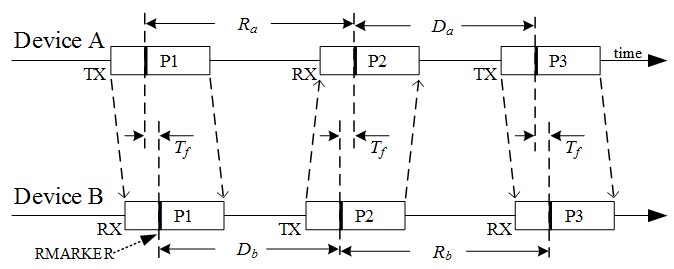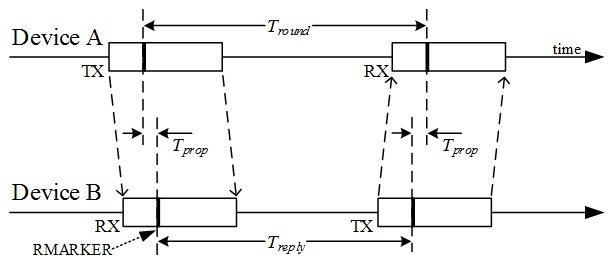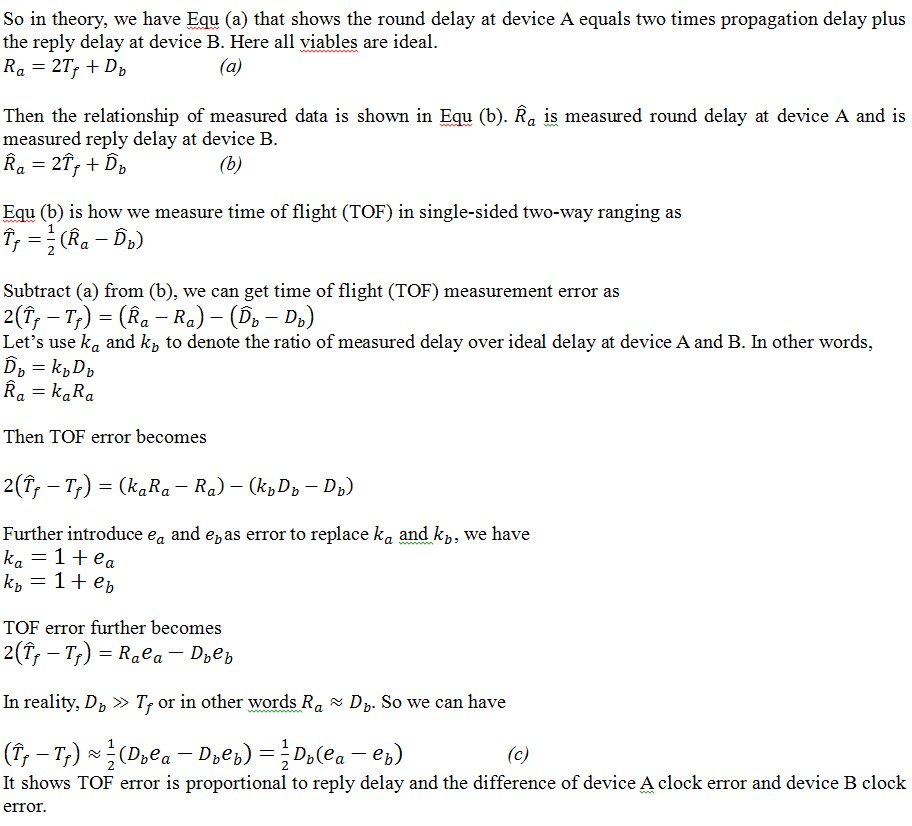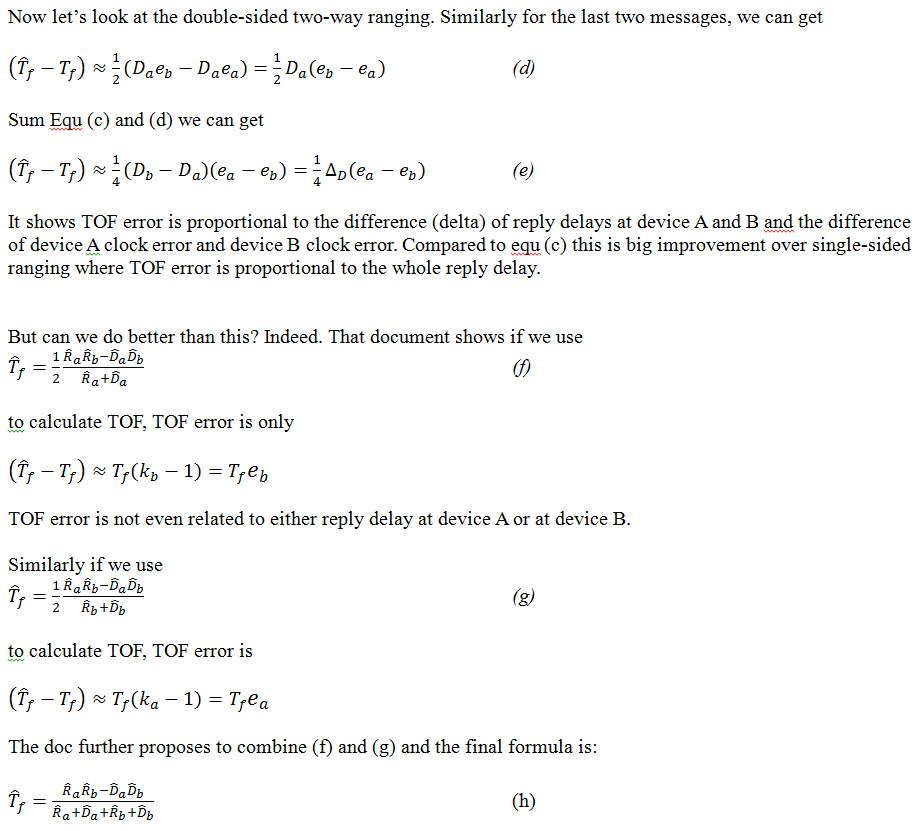# Single sided and double sided two way ranging formula and error

Make it to the Right and Larger Audience

Blog

# Single sided and double sided two way ranging formula and error

IEEE P802.15 Wireless Personal Area Networks has discussion about single-sided and double-sided two-way ranging. Some derivations are given at the end of this doc to show estimation formula for time of flight (TOF) and its error. Here are some of my notes. If you fully understand the doc, you can skip this post. Otherwise this post may be helpful.Above double-sided two-way ranging diagram shows the two round trips of double sider ranging and defines the terms used for the round trip times, Ra and Rb, and the reply times, Da and Db, for the pair of devices, A and B, participating in the two way ranging exchange to measure the time of flight, Tf.

If we only look at the 1st two messages, A->B and B->A. It is the single-sided two-way ranging as show in below.To be consistent we use double-sided variables for our discussion.the doc mentions:

Whether a system should use formula (f) or formula (g) would depend on whether it expects which clock it expects to be more accurate. For example, if the system knows B has a higher accuracy clocks then it should use formula (f).  If it expects neither to be more accurate than the other and it is more accurate to use the average result from (f) and (g) since this will always be as good as, or better than, the worst of the two (f) and (g).

the average result from (f) and (g) will be (h). The doc further states the TOF error calculated using (h) can be simplified as Tf(ea+eb)/2.

As mentioned above, TOF error of (f), (g), and (h) are not related to reply delay of device A or B. For double-sided two-way ranging it is generally suggested that the delay times of device A and B have to be nearly equal for the overall error to be acceptably small, but this is not a restriction when employing equations (f), (g) and (h), that burden is removed, so it is NOT required the use of the same response time at each end.  This gives much more implementation flexibility within the individual nodes participating in a ranging exchange and also facilitates more efficient implementations when a group of N nodes want to find the ½·N·(N-1) distances between each other.  Here, instead of separated ranging exchanges, messages can be combined, (e.g. a response from node B to Node A could also act as a ranging initiation message other nodes), to do the distance measurements with a much reduced over the air traffic, perhaps as few as 2·N messages.Author brief is empty
Groups:

Tags: